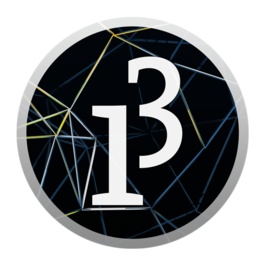Processing (programming language) Processing is an open source programming language and integrated development environment (IDE) built for the electronic arts, new media art, and visual design communities with the purpose of teaching the fundamentals of computer programming in a visual context, and to serve as the foundation for electronic sketchbooks. The project was initiated in 2001 by Casey Reas and Benjamin Fry, both formerly of the Aesthetics and Computation Group at the MIT Media Lab. One of the stated aims of Processing is to act as a tool to get non-programmers started with programming through the instant gratification of visual feedback. The language builds on the Java language, but uses a simplified syntax and graphics programming model. In 2012, they started the Processing Foundation along with Daniel Shiffman, who formally joined as a third project lead.

###### Using Processing¶

Note

We wont go in depth into programming with Processing. This here are just an example on how to export usable 3D data for our purpose.

As an example we have two simple 3D scene sketches that will create a .obj files you can use in Slicer or Paperkura. Before trying to create plans from the export you should take a look at the output in Blender. There might be some errors or problems with the sizes or centers of these models. To use these sketches you need to install the OBJExport library from Nervous System. There is a guide on the Processing Wiki on how to install libraries manually. If this worked you can open the Processing IDE, paste the sketches blow into the window and run them. The first sketch will create a simple scene with a cube and plane and will create the output.obj file next to your Processing sketch. The second sketch is a bit more complex. It creates several boxes and also does the output to a .obj file. These files can be imported and edited in Blender.

``````/**
* Simple sketch for doing papercraft
* uses the OBJExport library from
* http://n-e-r-v-o-u-s.com/tools/obj/
*
* @author Fabian "fabiantheblind" Morón Zirfas
*/
import nervoussystem.obj.*; // import the lib

/**
* the setup runs once.
* We only set the size of the canvas.
*
*/
void setup() {
size(500, 400, P3D);
}
/**
* This runs all the time. Actually we use the noLoop() statement.
* it prevents that our scene gets exported each frame.
*
*/
void draw() {
background(255);
// set the position of the camera
camera(width/10, height/3, -30, width/2, height/2, -250, 0, 1, 0);
// start the export
beginRecord("nervoussystem.obj.OBJExport", "output.obj");
// offset the scene to the center of the canvas.
// This makes the calculation of the coordiantes easier
translate(width/2, height/2, -250);
// make a transformation that only affects the plane
pushMatrix();
// rotate by 90 degrees on the x axis
// start the shape.
// write the vertices
vertex(-100, -100, -10);
vertex(100, -100, -10);
vertex(100, 100, -10);
vertex(-100, 100, -10);
// end the shape
endShape();
// reset the matrix of the the sketch so the
// rotation does not affect the other objects
popMatrix();
// we don't want the cube tu be solid
noFill();
// start the cube
vertex(-10, 10, 10);
vertex( 10, 10, 10);
vertex( 10, -10, 10);
vertex(-10, -10, 10);

vertex( 10, 10, 10);
vertex( 10, 10, -10);
vertex( 10, -10, -10);
vertex( 10, -10, 10);

vertex( 10, 10, -10);
vertex(-10, 10, -10);
vertex(-10, -10, -10);
vertex( 10, -10, -10);

vertex(-10, 10, -10);
vertex(-10, 10, 10);
vertex(-10, -10, 10);
vertex(-10, -10, -10);

vertex(-10, 10, -10);
vertex( 10, 10, -10);
vertex( 10, 10, 10);
vertex(-10, 10, 10);

vertex(-10, -10, -10);
vertex( 10, -10, -10);
vertex( 10, -10, 10);
vertex(-10, -10, 10);
// end the cube
endShape();
// end the recording
// this also exports the obj file
endRecord();
// we don't want to run the sketch again
noLoop();
}
``````
``````/**
* creates a scene with some boxes
*/
import nervoussystem.obj.*; // import the lib

void setup() {
size(500, 500, P3D);
}

void draw() {
background(0);
camera(280, 290, -44, 250, 250, 10, 0, 1, 0);
beginRecord("nervoussystem.obj.OBJExport", "output.obj");

for (int r = 0; r <= 360; r+=36) {
float x = cos(r) * 18;
float y = sin(r) * -12;
Box b = new Box(width/2 + x, height/2 + y);
b.rx = r;
b.ry = 30;
b.rz = 42;
b.draw();
}
endRecord();
noLoop();
}

class Box {
float d = 10;
float h = 10;
float w = 10;
float x = 0;
float y = 0;
float z = 0;
float rx = 0;
float ry = 0;
float rz = 0;
Box() {
}
Box(float x, float y) {
this.x = x;
this.y = y;
}
Box(float x, float y, float z) {
this.x = x;
this.y = y;
this.z = z;
}
Box(float x, float y, float z, float w, float h, float d) {
this.x = x;
this.y = y;
this.z = z;
this.d = d;
this.h = h;
this.w = w;
}

void draw() {
pushMatrix();
translate(this.x, this.y, this.z);